# 10.8 Vectors  (Page 11/22)

 Page 11 / 22

For the following exercises, evaluate each root.

Evaluate the cube root of $\text{\hspace{0.17em}}z\text{\hspace{0.17em}}$ when $\text{\hspace{0.17em}}z=64\mathrm{cis}\left(210°\right).$

Evaluate the square root of $\text{\hspace{0.17em}}z\text{\hspace{0.17em}}$ when $\text{\hspace{0.17em}}z=25\mathrm{cis}\left(\frac{3\pi }{2}\right).$

$5\mathrm{cis}\left(\frac{3\pi }{4}\right),5\mathrm{cis}\left(\frac{7\pi }{4}\right)$

For the following exercises, plot the complex number in the complex plane.

$6-2i$

$-1+3i$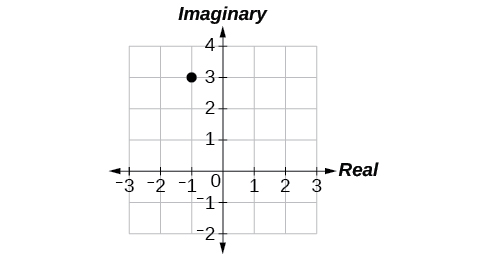## Parametric Equations

For the following exercises, eliminate the parameter $\text{\hspace{0.17em}}t\text{\hspace{0.17em}}$ to rewrite the parametric equation as a Cartesian equation.

$\left\{\begin{array}{l}x\left(t\right)=3t-1\hfill \\ y\left(t\right)=\sqrt{t}\hfill \end{array}$

${x}^{2}+\frac{1}{2}y=1$

Parameterize (write a parametric equation for) each Cartesian equation by using $\text{\hspace{0.17em}}x\left(t\right)=a\mathrm{cos}\text{\hspace{0.17em}}t\text{\hspace{0.17em}}$ and $\text{\hspace{0.17em}}y\left(t\right)=b\mathrm{sin}\text{\hspace{0.17em}}t\text{\hspace{0.17em}}$ for $\text{\hspace{0.17em}}\frac{{x}^{2}}{25}+\frac{{y}^{2}}{16}=1.$

Parameterize the line from $\text{\hspace{0.17em}}\left(-2,3\right)\text{\hspace{0.17em}}$ to $\text{\hspace{0.17em}}\left(4,7\right)\text{\hspace{0.17em}}$ so that the line is at $\text{\hspace{0.17em}}\left(-2,3\right)\text{\hspace{0.17em}}$ at $\text{\hspace{0.17em}}t=0\text{\hspace{0.17em}}$ and $\text{\hspace{0.17em}}\left(4,7\right)\text{\hspace{0.17em}}$ at $\text{\hspace{0.17em}}t=1.$

$\left\{\begin{array}{l}x\left(t\right)=-2+6t\hfill \\ y\left(t\right)=3+4t\hfill \end{array}$

## Parametric Equations: Graphs

For the following exercises, make a table of values for each set of parametric equations, graph the equations, and include an orientation; then write the Cartesian equation.

$\left\{\begin{array}{l}x\left(t\right)=3{t}^{2}\hfill \\ y\left(t\right)=2t-1\hfill \end{array}$

$\left\{\begin{array}{l}x\left(t\right)={e}^{t}\hfill \\ y\left(t\right)=-2{e}^{5\text{\hspace{0.17em}}t}\hfill \end{array}$

$y=-2{x}^{5}$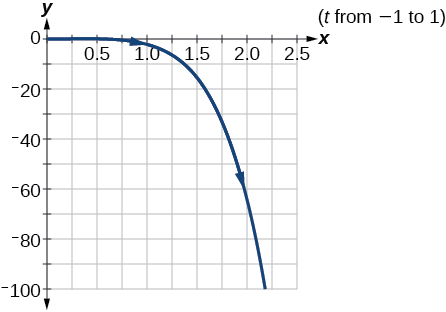$\left\{\begin{array}{l}x\left(t\right)=3\mathrm{cos}\text{\hspace{0.17em}}t\hfill \\ y\left(t\right)=2\mathrm{sin}\text{\hspace{0.17em}}t\hfill \end{array}$

A ball is launched with an initial velocity of 80 feet per second at an angle of 40° to the horizontal. The ball is released at a height of 4 feet above the ground.

1. Find the parametric equations to model the path of the ball.
2. Where is the ball after 3 seconds?
3. How long is the ball in the air?
1. $\left\{\begin{array}{l}x\left(t\right)=\left(80\mathrm{cos}\left(40°\right)\right)t\\ y\left(t\right)=-16{t}^{2}+\left(80\mathrm{sin}\left(40°\right)\right)t+4\end{array}$
2. The ball is 14 feet high and 184 feet from where it was launched.
3. 3.3 seconds

## Vectors

For the following exercises, determine whether the two vectors, $\text{\hspace{0.17em}}u\text{\hspace{0.17em}}$ and $\text{\hspace{0.17em}}v,\text{\hspace{0.17em}}$ are equal, where $\text{\hspace{0.17em}}u\text{\hspace{0.17em}}$ has an initial point $\text{\hspace{0.17em}}{P}_{1}\text{\hspace{0.17em}}$ and a terminal point $\text{\hspace{0.17em}}{P}_{2},\text{\hspace{0.17em}}$ and $\text{\hspace{0.17em}}v\text{\hspace{0.17em}}$ has an initial point $\text{\hspace{0.17em}}{P}_{3}\text{\hspace{0.17em}}$ and a terminal point $\text{\hspace{0.17em}}{P}_{4}.$

${P}_{1}=\left(-1,4\right),{P}_{2}=\left(3,1\right),{P}_{3}=\left(5,5\right)$ and $\text{\hspace{0.17em}}{P}_{4}=\left(9,2\right)$

${P}_{1}=\left(6,11\right),{P}_{2}=\left(-2,8\right),{P}_{3}=\left(0,-1\right)\text{\hspace{0.17em}}$ and $\text{\hspace{0.17em}}{P}_{4}=\left(-8,2\right)$

not equal

For the following exercises, use the vectors $\text{\hspace{0.17em}}u=2i-j\text{,}v=4i-3j\text{,}\text{\hspace{0.17em}}$ and $\text{\hspace{0.17em}}w=-2i+5j\text{\hspace{0.17em}}$ to evaluate the expression.

u v

2 v u + w

4 i

For the following exercises, find a unit vector in the same direction as the given vector.

a = 8 i − 6 j

b = −3 i j

$-\frac{3\sqrt{10}}{10}$ i $-\frac{\sqrt{10}}{10}$ j

For the following exercises, find the magnitude and direction of the vector.

$⟨6,-2⟩$

$⟨-3,-3⟩$

Magnitude: $\text{\hspace{0.17em}}3\sqrt{2},\text{\hspace{0.17em}}$ Direction: $\text{225°}$

For the following exercises, calculate $\text{\hspace{0.17em}}u\cdot v\text{.}$

u = −2 i + j and v = 3 i + 7 j

u = i + 4 j and v = 4 i + 3 j

$\text{16}$

Given v $=〈-3,4〉$ draw v , 2 v , and $\text{\hspace{0.17em}}\frac{1}{2}$ v .

Given the vectors shown in [link] , sketch u + v , u v and 3 v .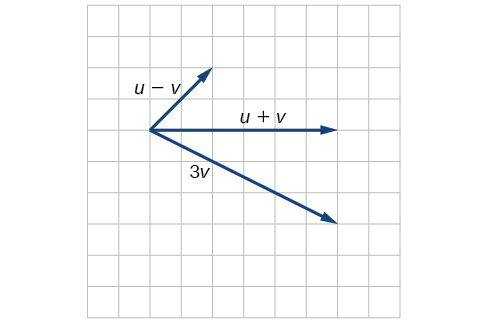Given initial point $\text{\hspace{0.17em}}{P}_{1}=\left(3,2\right)\text{\hspace{0.17em}}$ and terminal point $\text{\hspace{0.17em}}{P}_{2}=\left(-5,-1\right),\text{\hspace{0.17em}}$ write the vector $\text{\hspace{0.17em}}v\text{\hspace{0.17em}}$ in terms of $\text{\hspace{0.17em}}\text{\hspace{0.17em}}i\text{\hspace{0.17em}}\text{\hspace{0.17em}}$ and $\text{\hspace{0.17em}}j.\text{\hspace{0.17em}}$ Draw the points and the vector on the graph.

## Practice test

Assume $\text{\hspace{0.17em}}\alpha \text{\hspace{0.17em}}$ is opposite side $\text{\hspace{0.17em}}a,\beta \text{\hspace{0.17em}}$ is opposite side $\text{\hspace{0.17em}}b,\text{\hspace{0.17em}}$ and $\text{\hspace{0.17em}}\gamma \text{\hspace{0.17em}}$ is opposite side $\text{\hspace{0.17em}}c.\text{\hspace{0.17em}}$ Solve the triangle, if possible, and round each answer to the nearest tenth, given $\text{\hspace{0.17em}}\beta =68°,b=21,c=16.$

$\alpha =67.1°,\gamma =44.9°,a=20.9$

Find the area of the triangle in [link] . Round each answer to the nearest tenth.

A pilot flies in a straight path for 2 hours. He then makes a course correction, heading 15° to the right of his original course, and flies 1 hour in the new direction. If he maintains a constant speed of 575 miles per hour, how far is he from his starting position?

Convert $\text{\hspace{0.17em}}\left(2,2\right)\text{\hspace{0.17em}}$ to polar coordinates, and then plot the point.

Convert $\text{\hspace{0.17em}}\left(2,\frac{\pi }{3}\right)\text{\hspace{0.17em}}$ to rectangular coordinates.

$\left(1,\sqrt{3}\right)$

Convert the polar equation to a Cartesian equation: $\text{\hspace{0.17em}}{x}^{2}+{y}^{2}=5\mathrm{y.}$

Convert to rectangular form and graph: $r=-3\mathrm{csc}\text{\hspace{0.17em}}\theta .$

$y=-3$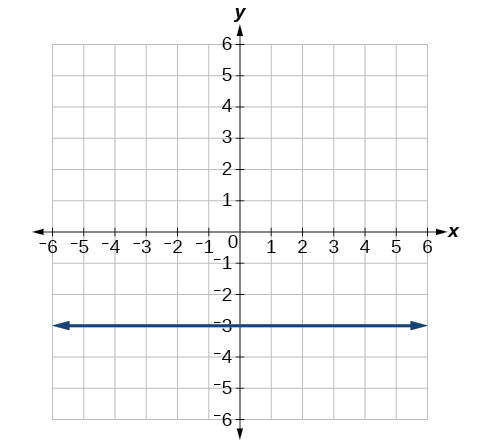Test the equation for symmetry: $\text{\hspace{0.17em}}r=-4\mathrm{sin}\left(2\theta \right).$

Graph $\text{\hspace{0.17em}}r=3+3\mathrm{cos}\text{\hspace{0.17em}}\theta .$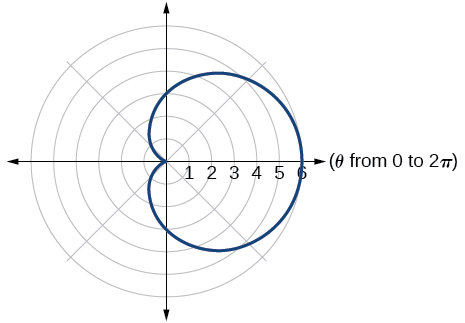Graph $\text{\hspace{0.17em}}r=3-5\text{sin}\text{\hspace{0.17em}}\theta .$

Find the absolute value of the complex number $5-9i.$

$\sqrt{106}$

Write the complex number in polar form: $\text{\hspace{0.17em}}4+i\text{.}$

Convert the complex number from polar to rectangular form: $\text{\hspace{0.17em}}z=5\text{cis}\left(\frac{2\pi }{3}\right).$

$\frac{-5}{2}+i\frac{5\sqrt{3}}{2}$

Given $\text{\hspace{0.17em}}{z}_{1}=8\mathrm{cis}\left(36°\right)\text{\hspace{0.17em}}$ and $\text{\hspace{0.17em}}{z}_{2}=2\mathrm{cis}\left(15°\right),$ evaluate each expression.

${z}_{1}{z}_{2}$

$\frac{{z}_{1}}{{z}_{2}}$

$4\mathrm{cis}\left(21°\right)$

${\left({z}_{2}\right)}^{3}$

$\sqrt{{z}_{1}}$

$2\sqrt{2}\mathrm{cis}\left(18°\right),2\sqrt{2}\mathrm{cis}\left(198°\right)$

Plot the complex number $\text{\hspace{0.17em}}-5-i\text{\hspace{0.17em}}$ in the complex plane.

Eliminate the parameter $\text{\hspace{0.17em}}t\text{\hspace{0.17em}}$ to rewrite the following parametric equations as a Cartesian equation: $\text{\hspace{0.17em}}\left\{\begin{array}{l}x\left(t\right)=t+1\hfill \\ y\left(t\right)=2{t}^{2}\hfill \end{array}.$

$y=2{\left(x-1\right)}^{2}$

Parameterize (write a parametric equation for) the following Cartesian equation by using $\text{\hspace{0.17em}}x\left(t\right)=a\mathrm{cos}\text{\hspace{0.17em}}t\text{\hspace{0.17em}}$ and $\text{\hspace{0.17em}}y\left(t\right)=b\mathrm{sin}\text{\hspace{0.17em}}t:$ $\frac{{x}^{2}}{36}+\frac{{y}^{2}}{100}=1.$

Graph the set of parametric equations and find the Cartesian equation: $\text{\hspace{0.17em}}\left\{\begin{array}{l}x\left(t\right)=-2\mathrm{sin}\text{\hspace{0.17em}}t\hfill \\ y\left(t\right)=5\mathrm{cos}\text{\hspace{0.17em}}t\hfill \end{array}.$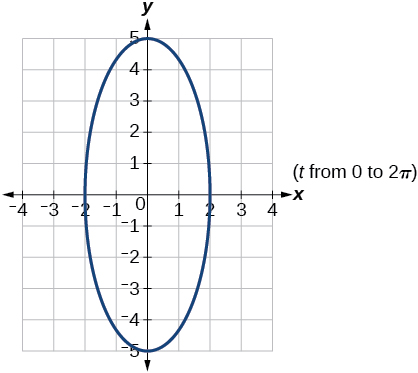A ball is launched with an initial velocity of 95 feet per second at an angle of 52° to the horizontal. The ball is released at a height of 3.5 feet above the ground.

1. Find the parametric equations to model the path of the ball.
2. Where is the ball after 2 seconds?
3. How long is the ball in the air?

For the following exercises, use the vectors u = i − 3 j and v = 2 i + 3 j .

Find 2 u − 3 v .

−4 i − 15 j

Calculate $\text{\hspace{0.17em}}u\cdot v.$

Find a unit vector in the same direction as $\text{\hspace{0.17em}}v.$

$\frac{2\sqrt{13}}{13}i+\frac{3\sqrt{13}}{13}j$

Given vector $\text{\hspace{0.17em}}v\text{\hspace{0.17em}}$ has an initial point $\text{\hspace{0.17em}}{P}_{1}=\left(2,2\right)\text{\hspace{0.17em}}$ and terminal point $\text{\hspace{0.17em}}{P}_{2}=\left(-1,0\right),\text{\hspace{0.17em}}$ write the vector $\text{\hspace{0.17em}}v\text{\hspace{0.17em}}$ in terms of $\text{\hspace{0.17em}}i\text{\hspace{0.17em}}$ and $\text{\hspace{0.17em}}j.\text{\hspace{0.17em}}$ On the graph, draw $\text{\hspace{0.17em}}v,\text{\hspace{0.17em}}$ and $\text{\hspace{0.17em}}-v.\text{\hspace{0.17em}}$

#### Questions & Answers

The sequence is {1,-1,1-1.....} has
amit Reply
circular region of radious
Kainat Reply
how can we solve this problem
Joel Reply
Sin(A+B) = sinBcosA+cosBsinA
Eseka Reply
Prove it
Eseka
Please prove it
Eseka
hi
Joel
June needs 45 gallons of punch. 2 different coolers. Bigger cooler is 5 times as large as smaller cooler. How many gallons in each cooler?
Arleathia Reply
find the sum of 28th term of the AP 3+10+17+---------
Prince Reply
I think you should say "28 terms" instead of "28th term"
Vedant
if sequence sn is a such that sn>0 for all n and lim sn=0than prove that lim (s1 s2............ sn) ke hole power n =n
SANDESH Reply
write down the polynomial function with root 1/3,2,-3 with solution
Gift Reply
if A and B are subspaces of V prove that (A+B)/B=A/(A-B)
Pream Reply
write down the value of each of the following in surd form a)cos(-65°) b)sin(-180°)c)tan(225°)d)tan(135°)
Oroke Reply
Prove that (sinA/1-cosA - 1-cosA/sinA) (cosA/1-sinA - 1-sinA/cosA) = 4
kiruba Reply
what is the answer to dividing negative index
Morosi Reply
In a triangle ABC prove that. (b+c)cosA+(c+a)cosB+(a+b)cisC=a+b+c.
Shivam Reply
give me the waec 2019 questions
Aaron Reply
the polar co-ordinate of the point (-1, -1)
Sumit Reply

### Read also:

#### Get the best Algebra and trigonometry course in your pocket!

Source:  OpenStax, Algebra and trigonometry. OpenStax CNX. Nov 14, 2016 Download for free at https://legacy.cnx.org/content/col11758/1.6
Google Play and the Google Play logo are trademarks of Google Inc.

Notification Switch

Would you like to follow the 'Algebra and trigonometry' conversation and receive update notifications?ByBy